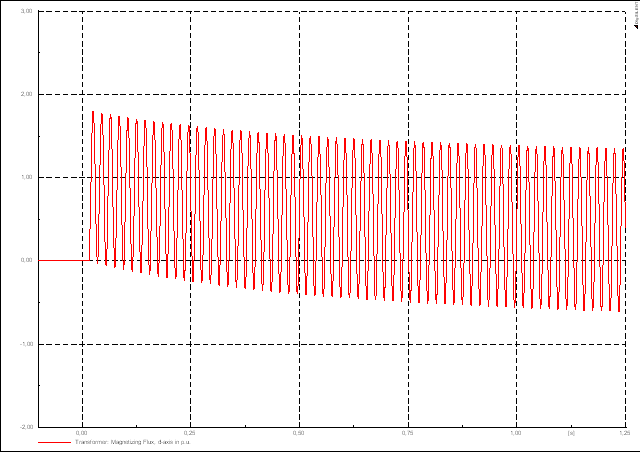# Transformer Inrush

(diff) ← Older revision | Latest revision (diff) | Newer revision → (diff)

Transformer (magnetising) inrush currents are caused by sudden step-changes in the transformer magnetising voltage and can be divided into three general types:

• Energisation inrush - occurs when a transformer is switched on from a de-energised state
• Recovery inrush - occurs when voltage is restored to the transformer after a brief voltage dip or interruption
• Sympathetic inrush - occurs when a transformer is switched on and is connected in parallel to an already energised transformer. The abrupt voltage drop caused by energising the first transformer can cause inrush currents (in the opposite direction) in the already energised transformer.

Transformer energisation is considered to cause the highest inrush currents and will be the focus of this article. When energised, an initial magnetising inrush current flows into the transformer. Typically, the inrush current lasts in the order of 0.1s and has the following magnitude:

• For transformers up to 2500kVA: 8x nominal current
• For transformers greater than 2500kVA: 10x nominal current

Transformer inrush currents are predominantly due to saturation of the transformer core and can be modelled as an RL switching transient with a saturable inductance L.

## Technical Background

Consider the application of a sinusoidal voltage to a transformer that is modelled very simply as a coil of N turns. By Faraday's law, we get:

$V_{p}\sin(\omega t+\theta )=N{\frac {d\phi }{dt}}\,$Where $V_{p}\,$is the voltage amplitude (V)

$\theta \,$is an arbitrary switching angle (radians)
$N\,$is the number of turns in the transformer coil
$\phi \,$is the transformer magnetising flux

To solve this differential equation for flux given some initial (residual) flux $\phi (0)\,$, we take the Laplace transform of it:

$V_{p}\left[{\frac {s\sin \theta +\omega \cos \theta }{s^{2}+\omega ^{2}}}\right]=N\left[s\phi (s)-\phi (0)\right]\,$Solving for $\phi (s)\,$:

$\phi (s)={\frac {V_{p}}{N}}\left[{\frac {s\sin \theta +\omega \cos \theta }{s(s^{2}+\omega ^{2})}}\right]+{\frac {\phi (0)}{s}}\,$Simplifying further:

$\phi (s)={\frac {V_{p}}{N}}\left[{\frac {\sin \theta }{s^{2}+\omega ^{2}}}+{\frac {\cos \theta }{\omega }}\left({\frac {1}{s}}-{\frac {s}{s^{2}+\omega ^{2}}}\right)\right]+{\frac {\phi (0)}{s}}\,$Taking the inverse Laplace transform, we finally get:

$\phi (t)={\frac {V_{p}}{\omega N}}\left[\sin \theta \sin(\omega t)+\cos \theta (1-\cos \omega t)\right]+\phi (0)\,$(1)

While this is a gross simplification of reality, the equation above can give us some intuition about the nature of the transformer flux during a transient switching on (energisation) event.

### Effects of Switching Angle

From equation (1) above, it can be shown that the switching angle results in a dc offset of the flux waveform. The figure below shows the effects of the switching angle on the flux waveform at three angles (relative to the ac supply voltage): 1) a positive zero crossing ($\theta =0$), 2) a voltage peak ($\theta ={\frac {\pi }{2}}$) and 3) a negative zero crossing ($\theta =\pi$).

Here it can be seen that switching at a positive zero crossing shifts the flux waveform up to a peak flux of 2pu (and vice versa for a negative zero crossing, i.e. peak flux down to -2pu). There is no dc offset when switching at a voltage peak. Any other switching angle will result in a dc offset in between the zero crossing waveforms.

### Effects of Residual Flux

It can be readily seen from transient flux waveform in equation (1) that the residual flux causes a dc offset in the flux waveform, i.e. a positive residual flux offsets the waveform up and vice versa for a negative residual flux.

Therefore, depending on the switching angle, a residual flux can actually be beneficial to keeping inrush currents low. For example, if the switching angle was a negative zero crossing (e.g. $\theta =\pi$, this would offset the flux waveform down. But a positive residual flux would have the opposite effect by offsetting the flux waveform up.

On the other hand, the residual flux can reinforce the dc offset caused by the switching angle and can result in peak flux values (absolute) well over 2pu.

### Transient Flux in Practical Transformers

In our idealised model above, the transient flux waveform on switching has constant dc offsets depending on the switching angle and residual flux. However in a practical transformer, the effect of leakage impedances (i.e. winding resistances and leakage reactances) will lead to an exponentially decaying dc offset component (like in the switching of RL circuits), with the rate of decay depending on the time constant ${\frac {R}{L}}$.

The figure below presents a more realistic transient flux waveform on switching, displaying a decaying dc component:Figure 3. Example of transient flux waveform in practical transformers

### Non-Linear Relationship between Flux and Current

We saw above that during transient conditions, the magnetising flux can rise up over 2pu (depending on the switching angle and residual flux). The other key factor that leads to high inrush currents is the non-linear relationship between flux and current.

The transformer core exhibits saturation characteristics like the hysteresis loop in the figure shown on the right. Here it can be seen that at 1 pu flux, the core is already saturating and the slope of the curve begins to approache a horizontal line. Therefore, if the flux is increased further above 1 pu flux, the current drawn can be several orders of magnitude higher.

### Summary

Putting all the pieces together, the general intuition explaining transformer energisation inrush can be put as follows:

1) On the sudden application of a voltage to the transformer (i.e. circuit breaker is closed), a transient flux waveform is generated that, depending on the switching angle and residual flux, can reach a peak of over two times nominal flux.
2) The relationship between flux and current is highly non-linear. At higher than nominal flux, the core will begin to saturate and the related magnetising currents can get very high. This is equivalent to saying that as the core saturates, the magnetising reactance decreases significantly.